GMAT Permutations and Combinations

Permutations

A permutation is a possible order in which to put a set of objects.  Suppose I had a shelf of 5 different books, and I wanted to know: in how many different orders can I put these 5 books?  Another way to say that is: 5 books have how many different permutations?

In order to answer this question, we need an odd math symbol: the factorial.  It’s written as an exclamation sign, and it means: the product of that number and all the positive integers below it, down to 1.  For example, 4! (read “four factorial”) is

4! = (4)(3)(2)(1) = 24

Here’s the permutation formula:

# of permutations of n objects = n!

So, five books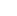the number of permutations is 5! = (5)(4)(3)(2)(1) = 120

Combinations

A combination is a selection from a larger set.  Suppose there is a class of 20, and we are going to pick a team of three people at random, and we want to know: how many different possible three-person teams could we pick?  Another way to say that is: how many different combinations of 3 can be taken from a set of 20?

This formula is scary looking, but really not bad at all.  If n is the size of the larger collection, and r is the number of elements that will be selected, then the number of combinations is given by

# of combinations =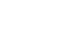Again, this looks complicated, but it gets simple very fast.  In the question just posed, n = 20, r = 3, and n – r = 17.  Therefore,

# of combinations =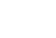To simplify this, consider that:

20! = (20)(19)(18)(17)(the product of all the numbers less than 17)

Or, in other words,

20! = (20)(19)(18)(17!)

That neat little trick allow us to enormously simplify the combinations formula:

# of combinations =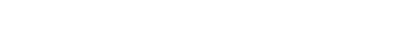That example is most likely harder than anything you’ll see on the GMAT math, but you may be asked to find combinations with smaller numbers.

Practice Questions

1) A bookseller has two display windows.  She plans to display 4 new fiction books in the left window, and 3 new non-fiction books in the right window.  Assuming she can put the four fiction books in any order, and separately, the three non-fiction books in any order, how many total configurations will there be for the two display windows?

(A) 24
(B) 72
(C) 144
(D) 336
(E) 420

2) The county-mandated guidelines at a certain community college specify that for the introductory English class, the professor may choose one of three specified novels, and choose two from a list of 5 specified plays.  Thus, the reading list for this introductory class is guaranteed to have one novel and two plays.  How many different reading lists could a professor create within these parameters?

(A) 15
(B) 30
(C) 90
(D) 150
(E) 360

1) The left window will have permutations of the 4 fiction books, so the number of possibilities for that window is

permutations = 4! = (4)(3)(2)(1) = 24

The right window will have permutations of the 3 non-fiction books, so the number of possibilities for that window is

permutations = 3! = (3)(2)(1) = 6

Any of the 24 displays of the left window could be combined with any of the 6 displays of the right window, so the total number of configurations is 24*6 = 144

2) There are three possibilities for the novel.   With the plays, we are taken a combination of 2 from a set of 5n = 5, r = 2, n – r = 3

# of combinations =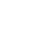=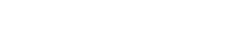If the plays are P, Q, R, S, and T, then the 10 sets of two are PQ, PR, PS, PT, QR, QS, QT, RS, RT, & ST.

Any of the three novels can be grouped with any of the 10 possible pairs of plays, for a total of 30 possible reading lists.

•